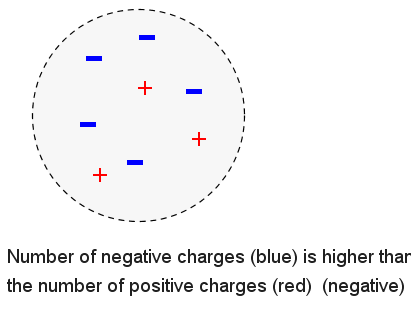# Electric Charges

Tutorials on electric charges with examples and explanations.

## Atoms

The atom is a basic unit of matter that consists of nucleus in the middle and a set of electrons surrounding the nucleus. The nucleus is made up of two types of particles: neutrons and protons.

Each electron has a negative electrical charge and each proton has a positive electrical charge. The charge of a proton and an electron are equal in magnitude but opposite in sign. Electrons and protons are electrically attracted to each other. Neutrons do not have a charge and are therefore electrically neutral.When an atom has the same number of electrons as there are protons within the nucleus, the net electrical charge of the atom is equal to zero.

If one or more electrons are added to an atom, the atom has more electrons that protons and becomes negatively charged. If an atom looses one or more electrons, the atom has more protons that electrons and becomes positively charged.

## Charged Objects

Because atoms are the basic unit of all matter, if the atoms of an object are negatively charged, then the object itself is negatively charged. If the atoms of an object are positively charged, then the object itself is positively charged. If an object has a balance of the two particles, it is considered neutral.

### Example 1

The object below has an equal number of positive and negative charges and is therefore electrically neutral.### Example 2

The object below has more negative charges that positive charges and is therefore electrically negative.### Example 3

The object below has more positive charges that negative charges and is therefore electrically positive.## Unit of Electric Charges

The SI unit of electric charge is the coulomb (C). Electric charges comes in small units called the elementary charge, e, approximately equal to 1.6�10-19 coulombs. The proton has a charge of e, and the electron has a charge of -e.
More To Explore